# Pulley Mass System | Definition, Examples in Physics – Laws of Motion

## Pulley Mass System | Definition, Examples in Physics – Laws of Motion

We are giving a detailed and clear sheet on all Physics Notes that are very useful to understand the Basic Physics Concepts.

Pulley Mass System Physics:
Determine the acceleration of the masses and the tension in the string.

(i) When unequal masses m1 and m2 are suspended from a pulley (m1 > m2)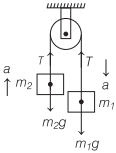m1g – T = m1a, and T – m2g = m2a
On solving equations, we get
$$a=\frac{\left(m_{1}-m_{2}\right)}{\left(m_{1}+m_{2}\right)} g,$$
$$T=\frac{2 m_{1} m_{2}}{\left(m_{1}+m_{2}\right)} g$$

(ii) When a body of mass m2 is placed on a frictionless horizontal surface, then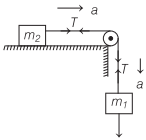Mass Pulley System acceleration, a = $$\frac{m_{1} g}{\left(m_{1}+m_{2}\right)}$$

Tension in a Pulley System with Two Masses, T = $$\frac{m_{1} m_{2} g}{\left(m_{1}+m_{2}\right)}$$

(iii) When a body of mass m2 is placed on a rough horizontal surface, then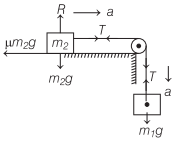Acceleration, a = $$\frac{\left(m_{1}-\mu m_{2}\right) g}{\left(m_{1}+m_{2}\right)}$$

Tension in string, T = $$\frac{m_{1} m_{2}(1+\mu) g}{\left(m_{1}+m_{2}\right)}$$

(iv) When two masses m1 and m2 (m1 > m2) are connected to a single mass M as shown in figure, then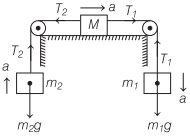m1g – T1 = m1a ……(i)
T2 – m2g = m2a ……(ii)
T1 – T2 = Ma ……(iii)

Acceleration, a = $$\frac{\left(m_{1}-m_{2}\right) g}{\left(m_{1}+m_{2}+M\right)}$$
Tension, T1 = $$\left(\frac{2 m_{2}+M}{m_{1}+m_{2}+M}\right) m_{1} g$$
T2 = $$\left(\frac{2 m_{1}+M}{m_{1}+m_{2}+M}\right) m_{2} g$$

(v) Motion on a smooth inclined plane, then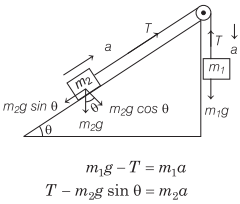m1g – T = m1a ……(i)
T – m2g sin θ = m2a ……(ii)
Acceleration, a = $$\left(\frac{m_{1}-m_{2} \sin \theta}{m_{1}+m_{2}}\right) g$$
Tension, T = $$\frac{m_{1} m_{2}(1+\sin \theta) g}{\left(m_{1}+m_{2}\right)}$$

(vi) Motion of two bodies placed on two inclined planes having different angle of inclination, thenAcceleration, a = $$\frac{\left(m_{1} \sin \theta_{1}-m_{2} \sin \theta_{2}\right) g}{m_{1}+m_{2}}$$
Tension, T = $$\frac{m_{1} m_{2}}{m_{1}+m_{2}}\left(\sin \theta_{1}+\sin \theta_{2}\right) g$$

Laws of Motion:
There are various laws in Physics that define the motion of the object. When an object is in motion whether it is linear or circular there is some force which is always imposed on it.